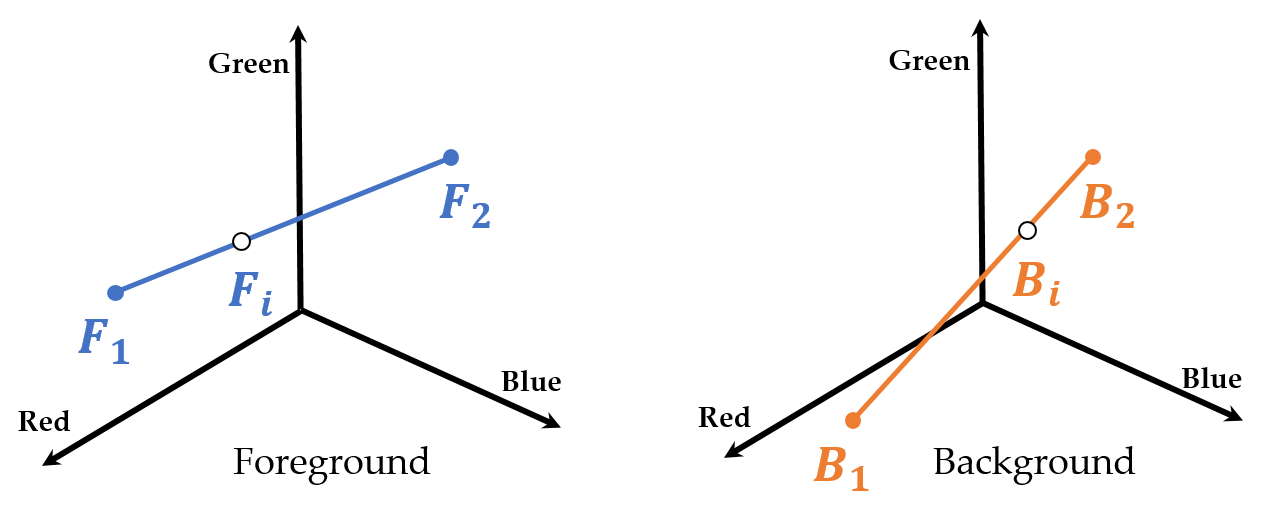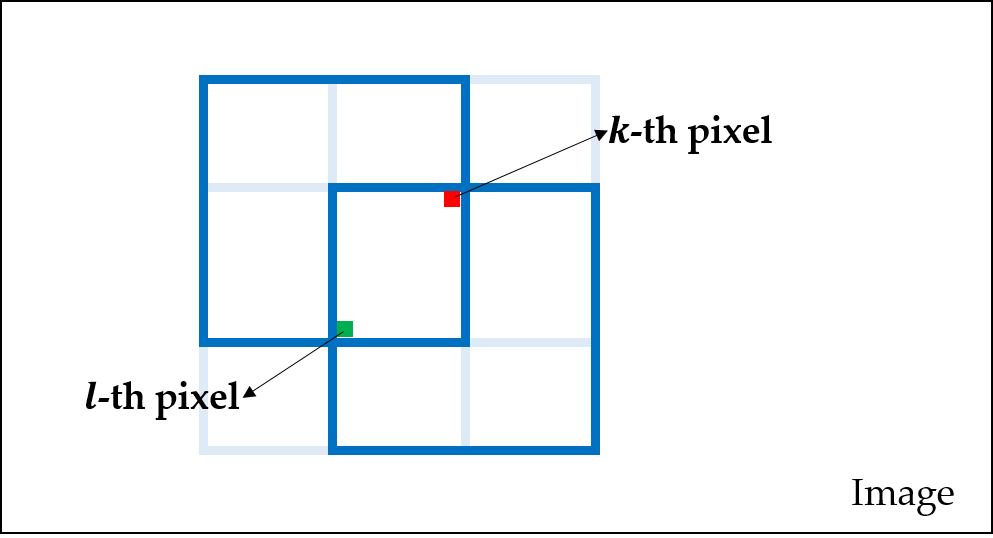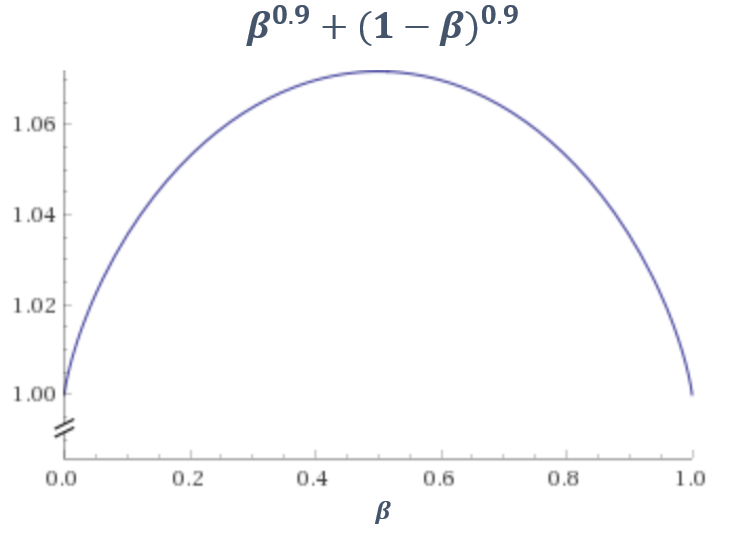# Matting Laplacian

## 1. Color Line Assumption• Matting is a technique that extracts the foreground from an image.
• Matting needs an assumption that for a small window $w$ of an image, its foreground pixels are colinear, so are its background pixels.
• More specifically, for a pixel $i \in w$, there exists $\beta_i$, $\gamma_i$, $F_1$, $F_2$, $B_1$, and $B_2$ such that $F_i = \beta_i F_1 + (1 - \beta_i) F_2$ and $B_i = \gamma_i B_1 + (1 - \gamma_i) B_2$ where $F_i$ is the foreground component of the pixel $i$ and $B_i$ is the background component of it.
• Moreover, the intensity $I_i$ of the pixel $i$ consists of $F_i$ and $B_i$ such that $I_i = \alpha_i F_i + (1 - \alpha_i) B_i$ for some $\alpha_i$.
• $I_i = \alpha_i (\beta_i F_1 + (1 - \beta_i) F_2) + (1 - \alpha_i)(\gamma_i B_1 + (1 - \gamma_i) B_2)$.
• It can be also represented as
$\begin{equation} \left(\begin{array}{ccc} F_2 - B_2 & F_1 - F_2 & B_1 - B_2 \end{array}\right) \left(\begin{array}{c} \alpha_i \\ \alpha_i \beta_i \\ (1 - \alpha_i) \gamma_i \end{array}\right) = I_i - B_2 \end{equation}$ $\begin{equation} \left(\begin{array}{c} \alpha_i \\ \alpha_i \beta_i \\ (1 - \alpha_i) \gamma_i \end{array}\right) = \left(\begin{array}{ccc} F_2 - B_2 & F_1 - F_2 & B_1 - B_2 \end{array}\right)^{-1} (I_i - B_2) = \left(\begin{array}{c} r^t_1 \\ r^t_2 \\ r^t_3 \end{array}\right) (I_i - B_2) \end{equation}$
• So $\alpha_i = r^t_1 I_i - r^t_1 B_2$. It implies that $\alpha_i$ and $I_i$ are in a linear relationship for $w$. It means that for every window $I_i$ and $\alpha_i$ are in an affine relationship.

## 2. Matting Laplaciain

• For the affinity of $I_i$ and $\alpha_i$, $\alpha_i \approx a I_i + b$ for some $a$ and $b$. Now consider the cost function $J(\alpha, a, b)$.
$\begin{equation} \sum_{j=1}^N \sum_{i \in w_j} (\alpha^j_i - (a_j I^j_i + b_j))^2 + \epsilon \Vert a_j \Vert^2_2 \end{equation}$

where $N$ is the number of windows in an image which is the same as the number of pixels in an image because the windows are created for each pixel. As the regularization term of this cost function, $\epsilon \Vert a_j \Vert^2_2$, is minimized, $a_j$ goes to zero, and it causes $a_i \approx b_j$. Here assume that $I_i$ is a scalar for simplicity, but it can be also extended to a three-dimensional vector.

• This cost function can be reformed as follows:
$\begin{equation} J(\alpha, a, b) = \sum_{j=1}^N \left\| \left(\begin{array}{cc} I^j_1 & 1 \\ \vdots & \vdots \\ I^j_W & 1 \\ \sqrt{\epsilon} & 0 \end{array}\right) \left(\begin{array}{c} a_j \\ b_j \end{array}\right) - \left(\begin{array}{c} \alpha^j_1 \\ \vdots \\ \alpha^j_W \\ 0 \end{array}\right) \right\|^2 = \sum_{j=1}^N \left\| G_j \left(\begin{array}{c} a_j \\ b_j \end{array}\right) - \overline{\alpha_j} \right\|^2 \end{equation}$

where $W$ is the number of pixels in each window.

• Let the optimal $a_j$ and $b_j$ for the window $w_j$ be $a^{*}_j$ and $b^{*}_j$, then from the normal equation
$\begin{equation} \left(\begin{array}{c} a^{*}_j \\ b^{*}_j \end{array}\right) = (G^t_j G_j)^{-1} G^t_j \overline{\alpha_j} \end{equation}$
• Now, the cost function $J(\alpha)$ for this optimal $a^{*}_j$ and $b^{*}_j$ is
$\begin{equation} J(\alpha) = \sum_{j=1}^N \left\| G_j \left(\begin{array}{c} a^{*}_j \\ b^{*}_j \end{array}\right) - \overline{\alpha_j} \right\|^2 = \sum_{j=1}^N \left\| G_j(G^t_j G_j)^{-1} G^t_j \overline{\alpha_j} - \overline{\alpha_j} \right\|^2 \end{equation}$

Let $G_j(G^t_j G_j)^{-1} G^t_j = X$, then $X^t = G_j(G^t_j G_j)^{-1} G^t_j = X$, so $X$ is symmetric. Moreover, $XX^t = XX = G_j(G^t_j G_j)^{-1} G^t_j G_j(G^t_j G_j)^{-1} G^t_j = G_j(G^t_j G_j)^{-1} G^t_j = X$. It implies that \begin{aligned} J(\alpha) &= \sum_{j=1}^N \left\| X \overline{\alpha_j} - \overline{\alpha_j} \right\|^2 = \sum_{j=1}^N \left\| (X - I) \overline{\alpha_j} \right\|^2 = \sum_{j=1}^N \overline{\alpha_j}^t (X - I)^t (X - I) \overline{\alpha_j} \\ &= \sum_{j=1}^N \overline{\alpha_j}^t (X^t - I) (X - I) \overline{\alpha_j} = \sum_{j=1}^N \overline{\alpha_j}^t (X^t X - X - X^t + I) \overline{\alpha_j} \\ &= \sum_{j=1}^N \overline{\alpha_j}^t (X - X - X^t + I) \overline{\alpha_j} = \sum_{j=1}^N \overline{\alpha_j}^t (I - X) \overline{\alpha_j} \\ &= \sum_{j=1}^N \overline{\alpha_j}^t (I - G_j(G^t_j G_j)^{-1} G^t_j) \overline{\alpha_j} = \sum_{j=1}^N \overline{\alpha_j}^t \overline{G_j} \overline{\alpha_j} \end{aligned}

• Besides, $G^t_j G_j$, $(G^t_j G_j)^{-1}$, $(G^t_j G_j)^{-1} G^t_j$, and $G_j(G^t_j G_j)^{-1} G^t_j$ are as follows:
\begin{aligned} G^t_j G_j &= \left(\begin{array}{cccc} I^j_1 & \cdots & I^j_W & \sqrt{\epsilon} \\ 1 & \cdots & 1 & 0 \end{array}\right) \left(\begin{array}{cc} I^j_1 & 1 \\ \vdots & \vdots \\ I^j_W & 1 \\ \sqrt{\epsilon} & 0 \end{array}\right) = \left(\begin{array}{cc} \epsilon + \sum_{i}^W I^2_i & \sum_{i}^W I_i \\ \sum_{i}^W I_i & W \end{array}\right) \\ &= \left(\begin{array}{cc} W(\sigma^2 + \mu^2) + \epsilon & W\mu \\ W\mu & W \end{array}\right) \\ (G^t_j G_j)^{-1} &= \frac{1}{W^2 (\sigma^2 + \mu^2) + W \epsilon - W^2 \mu^2} \left(\begin{array}{cc} W & -W\mu \\ -W\mu & W(\sigma^2 + \mu^2) + \epsilon \end{array}\right) \\ &= \frac{1}{W^2 \sigma^2 + W \epsilon} \left(\begin{array}{cc} W & -W\mu \\ -W\mu & W(\sigma^2 + \mu^2) + \epsilon \end{array}\right) \\ (G^t_j G_j)^{-1} G^t_j &= \frac{1}{W^2 \sigma^2 + W \epsilon} \left(\begin{array}{cc} W & -W\mu \\ -W\mu & W(\sigma^2 + \mu^2) + \epsilon \end{array}\right) \left(\begin{array}{cccc} I^j_1 & \cdots & I^j_W & \sqrt{\epsilon} \\ 1 & \cdots & 1 & 0 \end{array}\right) \\ &= \frac{1}{W \sigma^2 + \epsilon} \left(\begin{array}{cccc} I^j_1 - \mu & \cdots & I^j_W - \mu & \sqrt{\epsilon} \\ -\mu I^j_1 + \sigma^2 + \mu^2 + \frac{\epsilon}{W} & \cdots & -\mu I^j_W + \sigma^2 + \mu^2 + \frac{\epsilon}{W} & -\mu \sqrt{\epsilon} \end{array}\right) \\ \end{aligned} \begin{aligned} G_j(G^t_j G_j)^{-1} G^t_j &= \frac{1}{W \sigma^2 + \epsilon} \left(\begin{array}{cc} I^j_1 & 1 \\ \vdots & \vdots \\ I^j_W & 1 \\ \sqrt{\epsilon} & 0 \end{array}\right) \left(\begin{array}{cccc} I^j_1 - \mu & \cdots & I^j_W - \mu & \sqrt{\epsilon} \\ -\mu I^j_1 + \sigma^2 + \mu^2 + \frac{\epsilon}{W} & \cdots & -\mu I^j_W + \sigma^2 + \mu^2 + \frac{\epsilon}{W} & -\mu \sqrt{\epsilon} \end{array}\right) \\ &= \frac{1}{W \sigma^2 + \epsilon} \left(\begin{array}{cccc} \ddots & & & \sqrt{\epsilon} (I^j_1 - \mu) \\ & I_u I_v - I_u \mu + \sigma^2 + \mu^2 - I_v \mu + \frac{\epsilon}{W} & & \vdots \\ & & \ddots & \sqrt{\epsilon} (I^j_W - \mu) \\ \sqrt{\epsilon} (I^j_1 - \mu) & \cdots & \sqrt{\epsilon} (I^j_W - \mu) & \epsilon \end{array}\right) \end{aligned}

where $u$, $v \in [1, W]$ are integers. From equations as above, $(\overline{G_j}_{uv})$ which means the $(u, v)$ element of $\overline{G_j}$ is \begin{aligned} (\overline{G_j})_{uv} &= (I- G_j(G_j^t G_j)^{-1} G_j^t )_{uv} \\ &= \delta_{uv} - \frac{1}{W \sigma^2 + \epsilon} \left( I_u I_v - I_u \mu + \sigma^2 + \mu^2 - I_v \mu + \frac{\epsilon}{W} \right) \\ &= \delta_{uv} - \frac{1}{W \sigma^2 + \epsilon} \left( \sigma^2 + \frac{\epsilon}{W} + (I_u - \mu)(I_v - \mu) \right) \\ &= \delta_{uv} - \frac{1}{W} \left( 1 + \frac{1}{\sigma^2 + \frac{\epsilon}{W}}(I_u - \mu)(I_v - \mu) \right) \end{aligned}

where \delta_{uv} is the Kronecker delta which means that $1$ if $u = v$ and $0$ otherwise.

• Let the top-left $W \times W$ submatrix of $\overline{G_j}$ be $[\overline{G_j}]$. Now, $J(\alpha)$ can be represented as the form $m^t Lm$ where $L$ is the Matting Laplacian. Particularly, $m$ is an $N \times 1$ matte vector where $m_k$ is the matte of the $k$-th pixel. Therefore, there exist multiple $i$ and $j$ pairs such that $\alpha^j_i = m_k$.
\begin{aligned} J(\alpha) &= \sum_{j=1}^N \overline{\alpha_j}^t \overline{G_j} \overline{\alpha_j} = \sum_{j=1}^N \left(\begin{array}{cccc} \alpha^j_1 & \cdots & \alpha^j_W & 0 \end{array}\right) \overline{G_j} \left(\begin{array}{c} \alpha^j_1 \\ \vdots \\ \alpha^j_W \\ 0 \end{array}\right) \\ &= \sum_{j=1}^N \left(\begin{array}{ccc} \alpha^j_1 & \cdots & \alpha^j_W \end{array}\right) [\overline{G_j}] \left(\begin{array}{c} \alpha^j_1 \\ \vdots \\ \alpha^j_W \end{array}\right) = m^t L m\\ &= \left(\begin{array}{ccc} m_1 & \cdots & m_N \end{array}\right) \left(\begin{array}{ccc} L_{11} & \cdots & L_{1N} \\ \vdots & \ddots & \vdots \\ L_{N1} & \cdots & L_{NN} \end{array}\right) \left(\begin{array}{c} m_1 \\ \vdots \\ m_N \end{array}\right) \\ \\ &= \begin{array}{c} m_1 L_{11} m_1 + m_1 L_{12} m_2 + \cdots + m_1 L_{1N} m_N + \\ \cdots \\ m_i L_{i1} m_1 + m_i L_{i2} m_2 + \cdots + m_i L_{iN} m_N + \\ \cdots \\ m_N L_{N1} m_1 + m_N L_{N2} m_2 + \cdots + m_N L_{NN} m_N \end{array} \end{aligned}
• $L_{kl}$ appears in only $m_k L_{kl} m_l$ part. Therefore, $L_{kl}$ is the sum of the coefficients of $\alpha^{j_1}_{i_1} \alpha^{j_2}_{i_2}$ pairs which are equivalent to $m_k m_l$ in $\sum_{j=1}^N \overline{\alpha_j}^t \overline{G_j} \overline{\alpha_j}$.In other words, for the window-set $w_{kl}$ that includes the $k$-th and $l$-th pixels together in an image, $L_{kl}$ can be figured out from this by calculating $\sum_{w \in w_{kl}} \overline{\alpha_w}^t \overline{G_w} \overline{\alpha_w}$. This is because there exists the set $\Theta = \{ (p_w, q_w) \vert p_w = m_k, q_w = m_l, p_w, q_w \in \overline{\alpha_w} \text{ and } w \in w_{kl} \}$. Therefore, $L_{kl}$ is as follows: \begin{aligned} L_{kl} &= \sum_{w \in w_{kl}} (\overline{G_w})_{p_w q_w} = \sum_{w \in w_{kl}} \delta_{p_w q_w} - \frac{1}{W_{p_w q_w}} \left(1 + \frac{1}{\sigma^2_w + \frac{\epsilon}{W}}(I_{p_w} - \mu_w)(I_{q_w} - \mu_w)\right) \end{aligned}

• $L$ is symmetric since $L_{kl} = L_{lk}$.
• Finally, $J = m^t L m$, so it can be solved by solving $Lm = 0$ because $\frac{\partial J}{\partial m} = 2m^t L = 0$.

• Any constant $m$ is in nullspace of $L$. Consider $cL(1, \cdots, 1)^t$ for $c \in R$. Then its $i$-th row element is $\sum_{j=1}^N L_{ij}$. For the window-set $w_{ii}$ that includes the $i$-th pixel, let $p_w = m_i$, $w \in w_{ii}$. Then,
\begin{aligned} \sum_{j=1}^N L_{ij} &= \sum_{w \in w_{ii}} \sum_{u \in w} (\overline{G_w})_{p_w u} = \sum_{w \in w_{ii}} \sum_{u \in w} \delta_{p_w u} - \frac{1}{W} \left(1 + \frac{1}{\sigma^2_w + \frac{\epsilon}{W}}(I_{p_w} - \mu_w)(I_{u} - \mu_w)\right) \\ &= \sum_{w \in w_{ii}} \left( \sum_{u \in w} \delta_{p_w u} - \sum_{u \in w} \frac{1}{W} \left(1 + \frac{1}{\sigma^2_w + \frac{\epsilon}{W}}(I_{p_w} - \mu_w)(I_{u} - \mu_w)\right) \right) \\ &= \sum_{w \in w_{ii}} \left( 1 - 1 - \frac{1}{W \sigma^2_w + \epsilon} (I_{p_w u} - \mu_w) \sum_{u \in w} (I_{u} - \mu_w) \right) \\ &= \sum_{w \in w_{ii}} - \frac{1}{W \sigma^2_w + \epsilon} (I_{p_w u} - \mu_w) (W\mu_w - W\mu_w) \\ &= \sum_{w \in w_{ii}} 0 = 0 \end{aligned}
• So user scribbles are required which denote some of foreground and background pixels in an image. Let $U$ be a $N \times 1$ vector whose $i$-th element is $1$ if it is the known foreground pixel or $0$ otherwise. In addition, let $F = \{ f \vert U_f = 1 \}$.
\begin{aligned} J = \min m^t L m + \lambda \sum_{f \in F} (m_f - 1)^2 = \min m^t L m + \lambda (m - U)^t D (m - U) \end{aligned}

where $D$ is an $N \times N$ diagonal matrix which $D_{ii} = 1$ if $U_{i} = 1$ or $0$ otherwise.

• To solve, $J$, we need the following:
\begin{aligned} \frac{\partial J}{\partial m} &= 2 m^t L + \lambda (m - U)^t (D + D^t) = 2 m^t L + 2 \lambda (m^t - U^t) D \\ &= 2 m^t (L + \lambda D) - 2 \lambda U^t D = 0 \\ &\implies m^t (L + \lambda D) = \lambda U^t D = \lambda U^t \\ &\implies (L + \lambda D)^t m = (L + \lambda D) m = \lambda U \end{aligned}

Therefore, the solution can be from the linear system $(L + \lambda D)m = \lambda U$ where $\lambda$ is a large number, for example, $100$.

## 4. The eigenvectors of $L$

• Without user scribbles, the solution of $J = m^t L m$ is that of $Lm = 0$.
• Consider $Lv = \lambda v$ where $v$ is the eigenvector of $L$ and $\lambda$ is the eigenvalue of $v$. If we choose $\lambda$ closing to $0$, then $Lv = \lambda v \approx 0 = Lm$.
• This eigenvector $v$ looks like a grayscale image.
• Let the set $\Phi = \{ v \vert Lv = \lambda v, \lambda \approx 0 \}$. Then for $s = \vert \Phi \vert$, $Lv_1 + \cdots + Lv_s = L(v_1 + \cdots + v_s) \approx 0$. It means that any combination of elements in $\Phi$ can be a candidate of $m$. More formally, there exist some constants such that $m \approx \sum_{i=1}^s c_i v_i$ and $\sum_{i=1}^s c_i = 1$ for $c \in \R$. However, it is more realistic that we define an $N \times 1$ vector $\beta$ such that $m \approx \sum_{i=1}^s \beta_i v_i$ and $\sum_{i=1}^s \beta_{i, j} = 1$ where $\beta_{i, j}$ denotes the $j$-th element of $\beta_i$ for $1 \le j \le N$.
• Meanwhile, it is likely that most elements of $m$ are $0$ or $1$ in the ground truth of $m$. This is because most $m_i$ is in range $(0, 1)$ only when the $i$-th pixel is in the boundary of the foreground. So, it is reasonable that if $m$ is well estimated, most elements of $m$ are $0$ or $1$.• Therefore, $J$ can be reformed as follows:
\begin{aligned} J = \min \sum_{i=1}^s \sum_{j=1}^N \vert \beta_{i, j} \vert^\rho + \vert 1 - \beta_{i, j} \vert^\rho \end{aligned}

Each $\beta_{i}$ is forced to be distributed to $0$ or $1$ when it is minimized. This cost function produces a good solution even when user scribbles are not given.

## Reference

 Richard J. Radke, (2012) Computer Vision for Visual Effects, Cambridge University Press, Cambridge.